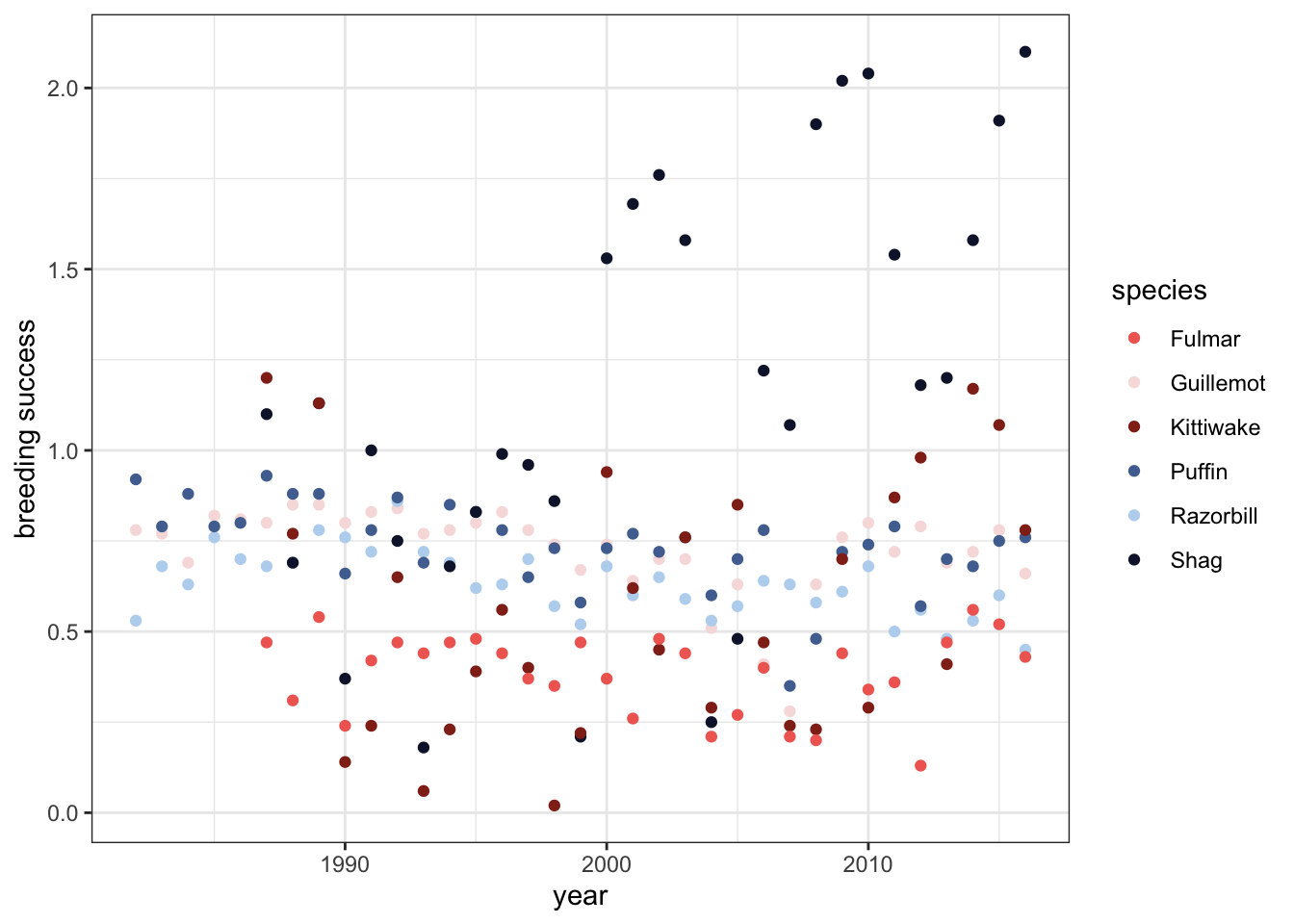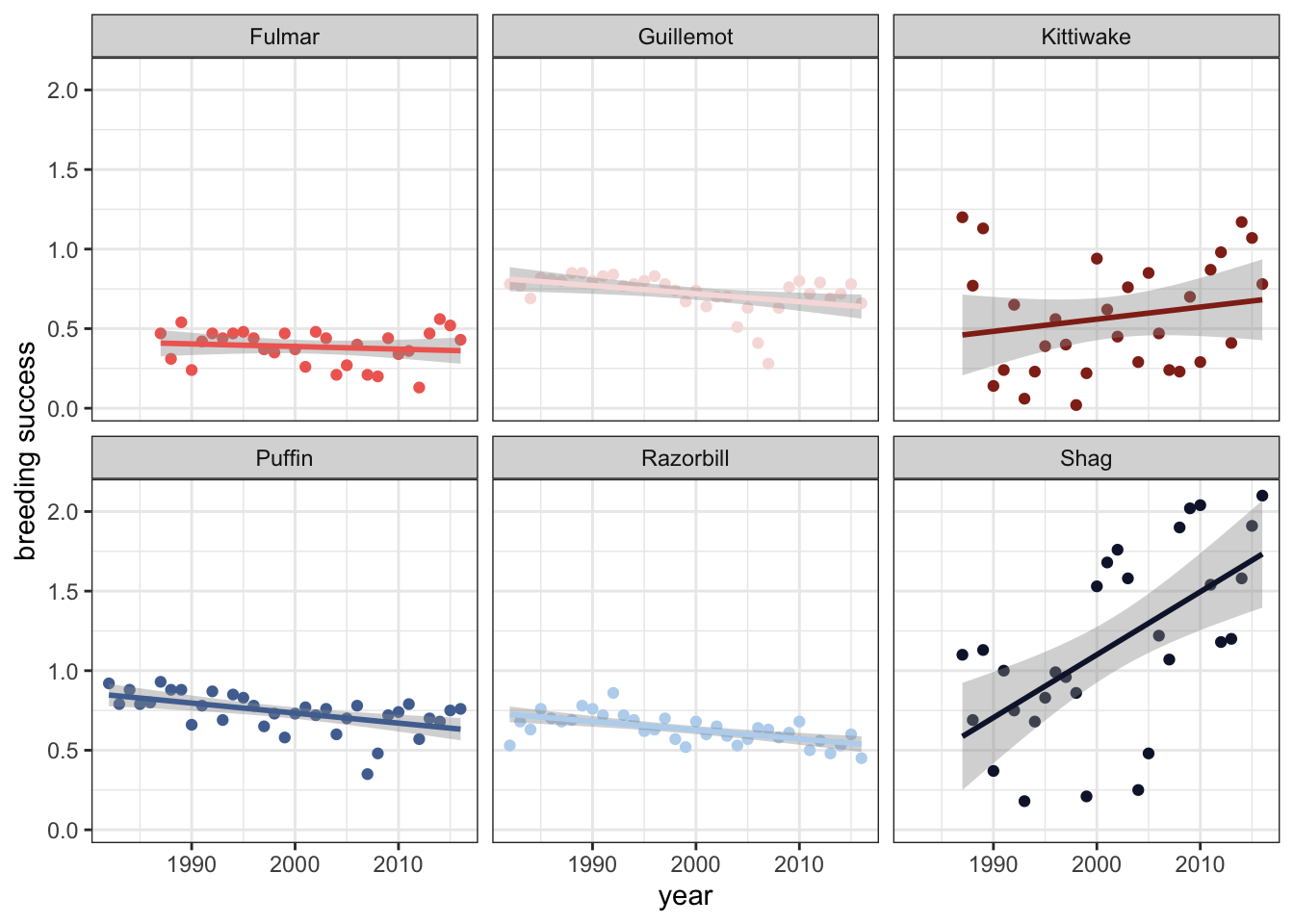Since 1982 the UK’s Centre for Ecology and Hydrology has been tracking the breeding habits of auks on the Isle of May, a small island east of Scotland. The auk family of sea birds, which includes the iconic puffin, live primarily on the coasts of the northern Atlantic Ocean, and climate change could have significant effects on their ability to breed. We’ll use the CEH’s collected data, plus weather and temperature data from the Meteorological Office, to see how various species of auk have fared over the course of this long-term study. We’ll use the {lme4} package to create random-intercept, mixed-effect linear models that account for the hierarchical structure of the data.

Throughout this report, I’ve included the code used in collapsible sections. For more insight into how I developed a particular solution, just click “code”.

#### Preparing the Data:

``````breeding <- read.csv("IMLOTSBSDataset1982-2016.csv")

# the data is in wide format, so we'll pivot it to make a "species" column,
#and use regex to assign new, accurate column names.
br <- breeding %>%
pivot_longer(
cols = 2:13,
names_to = c("species", "type"),
names_pattern = "([\\w]+)[.]([\\w]+)")

#now we'll pare it down to breeding success data:

success <- br %>%
filter(type == "BS")

#looks good!``````

Now we’ve arranged the data so we have each species’ rate of breeding success for each year of the study easily within reach. We’ll create a basic time series showing how each species’ breeding success has change over time. This will help us see patterns that might be useful for further study.

#### Visualizing breeding success over time:

``````birdpal <- c(Guillemot = "#F7DEDE", Razorbill = "#BAD5EE", Puffin = "#5071A0", Shag = "#111A36", Kittiwake = "#922A1C", Fulmar = "#F06A60")

ggplot(success, aes(x=year, y=value, color = species)) +
geom_point() +
theme_bw() +
scale_color_manual(values = birdpal) +
ylab("breeding success")````````````#faceted with lines of best fit:

ggplot(success, aes(x=year, y=value, color=species)) +
geom_point() +
facet_wrap(~species) +
geom_smooth(method = "lm") +
theme_bw() +
theme(legend.position = "none") +
scale_color_manual(values = birdpal) +
ylab("breeding success")``````
``FALSE `geom_smooth()` using formula 'y ~ x'``Our visualizations show a lot of variation from species to species! Shags and Kittiwakes appear to have increased breeding success over time, while the others are showing a mild decline. There’s also a lot of differences in error - how closely those data adhere to the basic linear pattern. To get more numbers in front of us, we’ll run a linear regression on each species in the dataset and collect that important info in a table.

#### Basic Linear Regressions by Species:

``````bird_list <- unique(br\$species)
t <- c("species", "slope", "conflo", "confhi", "r2", "adj r2", "pval")
r <- data.frame("species", "slope", "conflo", "confhi", "r2", "adj r2", "pval")
names(r) <- t

for (b in 1:length(bird_list)) {
bird <- bird_list[b]
df <- success %>%
filter(species == bird)
line <- lm(value ~ year, data = df)
slope <- round(tidy(line)[2,2],5)
conflo <- round(confint(line)[2,1],5)
confhi <- round(confint(line)[2,2],5)
r2 <- round(summary(line)\$r.squared,5)
pval <- round(tidy(line)[2,5],5)
newvector <- c(bird, slope, conflo, confhi, r2, r2a, pval)
names(newvector) <- t
r <- rbind(r, newvector)
}
r <- r[-1,]
r %>%
mutate(confhi = as.numeric(confhi),
conflo = as.numeric(conflo),
conf.range = (confhi-conflo))``````
``````FALSE     species    slope   conflo   confhi      r2   adj r2    pval conf.range
FALSE 1 Guillemot -0.00508 -0.00889 -0.00126 0.18137  0.15657 0.01075    0.00763
FALSE 2 Razorbill -0.00548 -0.00798 -0.00299 0.37659   0.3577   9e-05    0.00499
FALSE 3    Puffin -0.00631 -0.00986 -0.00276 0.28397  0.26228 0.00098    0.00710
FALSE 4      Shag  0.03953  0.01958  0.05948 0.37045  0.34796 0.00036    0.03990
FALSE 5 Kittiwake  0.00763 -0.00742  0.02268 0.03708  0.00269 0.30797    0.03010
FALSE 6    Fulmar -0.00164 -0.00652  0.00324  0.0166 -0.01852 0.49741    0.00976``````
``````#visualizing our basic linear models
ggplot(success, aes(x=year, y=value, color=species)) +
geom_point(alpha = .4) +
geom_smooth(method = "lm", se=TRUE, alpha=.2) +
theme_bw() +
scale_color_manual(values=birdpal) +
ylab("breeding success") +
theme(legend.position = c(.1,.70),
legend.text = element_text(size=8),
legend.title = element_text(size=9)) +
ggtitle("Linear models of breeding success by species")``````
``FALSE `geom_smooth()` using formula 'y ~ x'``